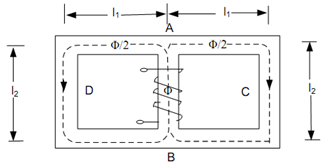## Parallel Magnetic Circuit Assignment Help

Assignment Help: >> ElectroMagnetism - Parallel Magnetic Circuit

Parallel Magnetic Circuit:

Parallel magnetic circuit contain two parallel magnetic paths acted upon by the same m.m.f. as illustrated in Figure. Here ACB and ADB are the two magnetic paths acted upon by the same m.m.f. Each of the magnetic path has an average length of 2 (l1 + l2).Figure: Parallel Magnetic Circuit